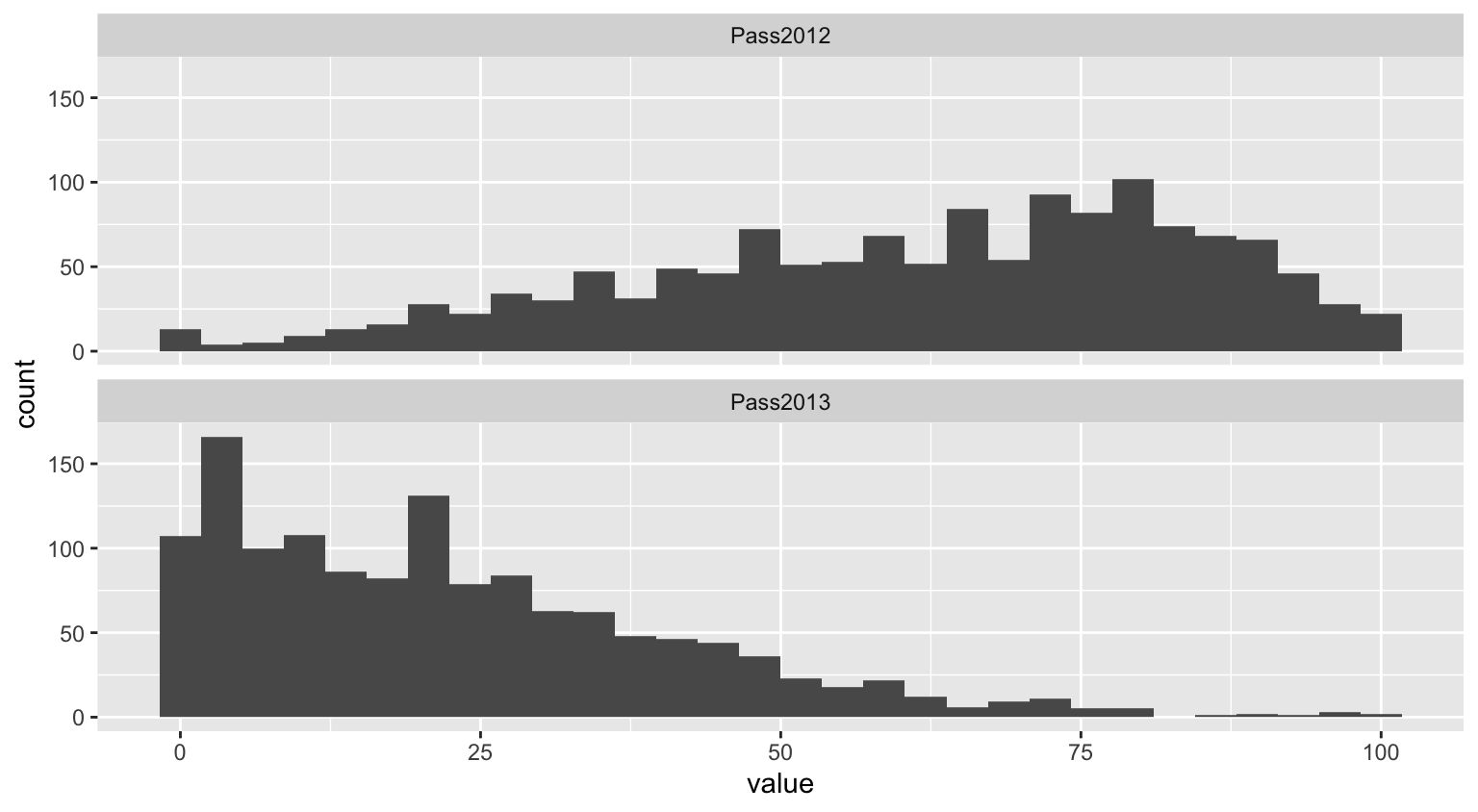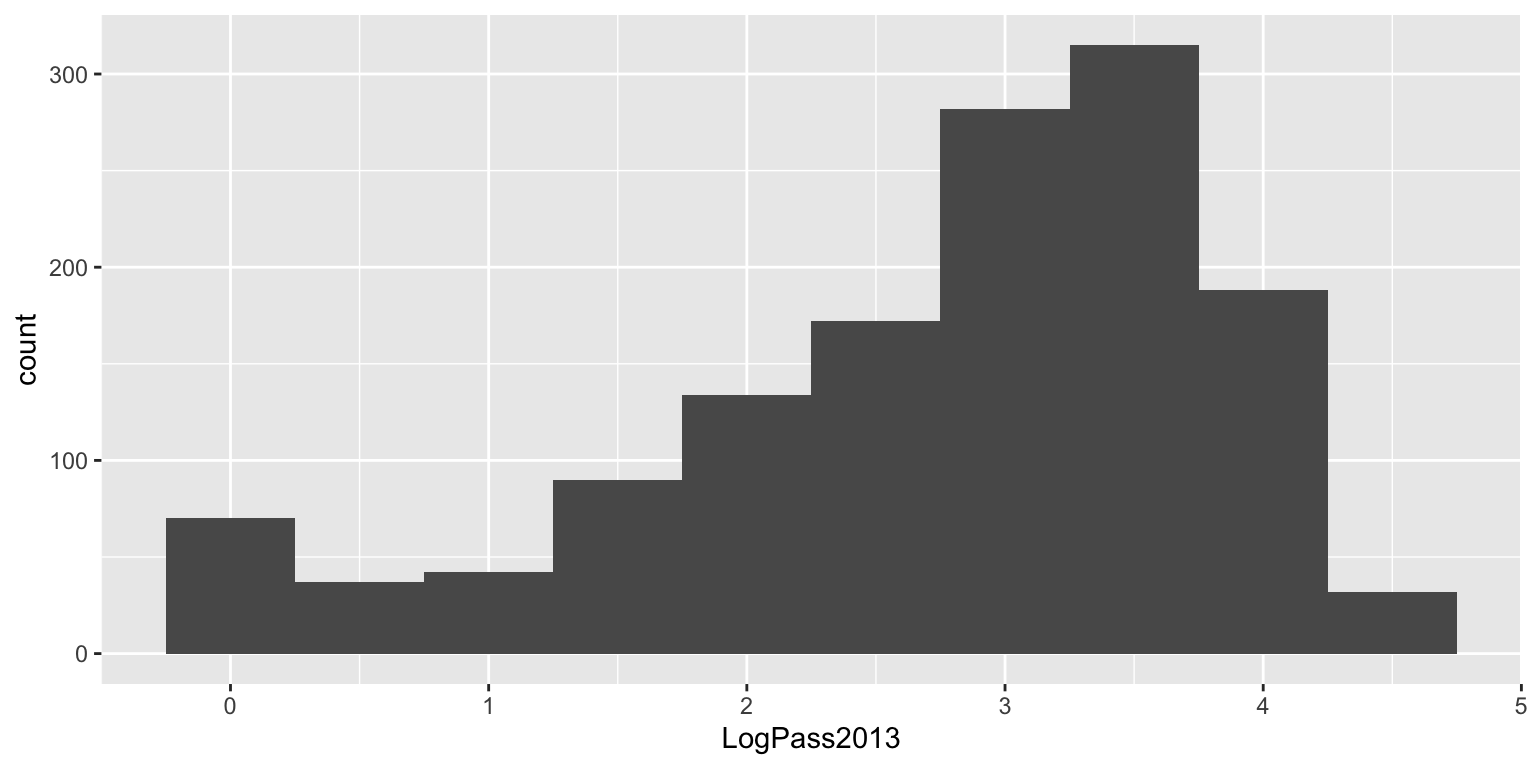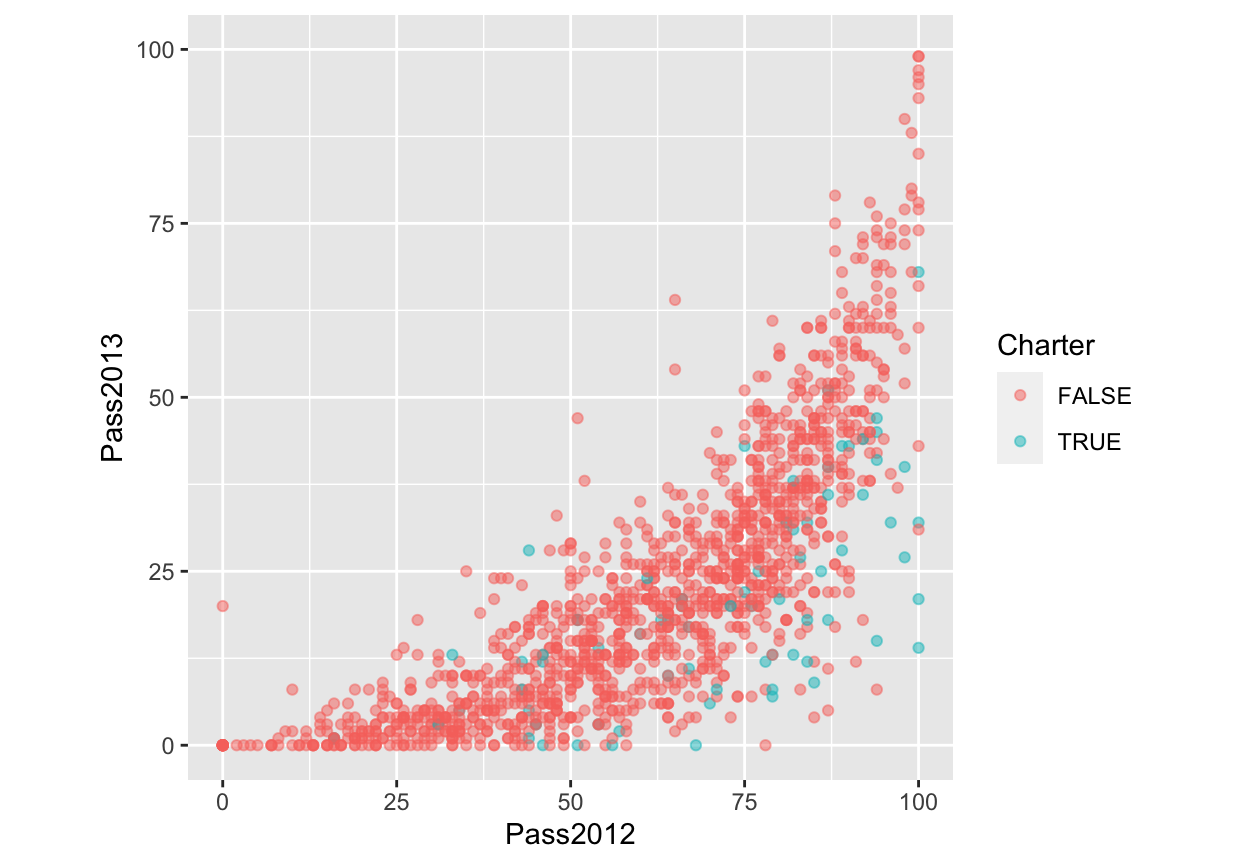April 1, 2020

## NYS Report Card

NYS publishes data for each school in the state. We will look at the grade 8 math scores for 2012 and 2013. 2013 was the first year the tests were aligned with the Common Core Standards. There was a lot of press about how the passing rates for most schools dropped. Two questions we wish to answer:

1. Did the passing rates drop in a predictable manner?
2. Were the drops different for charter and public schools?
```load('../course_data/NYSReportCard-Grade7Math.Rda')
```##       BEDSCODE                                  School NumTested2012 Mean2012 Pass2012 Charter
## 1 010100010020                    NORTH ALBANY ACADEMY            47      649       13   FALSE
## 2 010100010030         WILLIAM S HACKETT MIDDLE SCHOOL           212      652       30   FALSE
## 3 010100010045 STEPHEN AND HARRIET MYERS MIDDLE SCHOOL           262      670       50   FALSE
## 4 010100860867         KIPP TECH VALLEY CHARTER SCHOOL            61      684       85    TRUE
##   GradeSubject County                                BOCES NumTested2013 Mean2013 Pass2013

## Descriptive Statistics

`summary(reportCard\$Pass2012)`
```##    Min. 1st Qu.  Median    Mean 3rd Qu.    Max.
##    0.00   46.00   65.00   61.73   80.00  100.00```
`summary(reportCard\$Pass2013)`
```##    Min. 1st Qu.  Median    Mean 3rd Qu.    Max.
##    0.00    7.00   20.00   22.83   33.00   99.00```

## Histograms

```melted <- melt(reportCard[,c('Pass2012', 'Pass2013')])
ggplot(melted, aes(x=value)) + geom_histogram() + facet_wrap(~ variable, ncol=1)```## Log Transformation

Since the distribution of the 2013 passing rates is skewed, we can log transfor that variable to get a more reasonably normal distribution.

```reportCard\$LogPass2013 <- log(reportCard\$Pass2013 + 1)
ggplot(reportCard, aes(x=LogPass2013)) + geom_histogram(binwidth=0.5)```## Scatter Plot

```ggplot(reportCard, aes(x=Pass2012, y=Pass2013, color=Charter)) +
geom_point(alpha=0.5) + coord_equal() + ylim(c(0,100)) + xlim(c(0,100))```## Scatter Plot (log transform)

```ggplot(reportCard, aes(x=Pass2012, y=LogPass2013, color=Charter)) +
geom_point(alpha=0.5) + xlim(c(0,100)) + ylim(c(0, log(101)))```